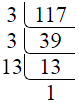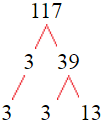# Factors of 117The factors of 117 are 1, 3, 9, 13, 39, and 117 i.e. F117 = {1, 3, 9, 13, 39, 117}. The factors of 117 are all the numbers that can divide 117 without leaving a remainder.

We can check if these numbers are factors of 117 by dividing 117 by each of them. If the result is a whole number, then the number is a factor of 117. Let's do this for each of the numbers listed above:

·        1 is a factor of 117 because 117 divided by 1 is 117.

·        3 is a factor of 117 because 117 divided by 3 is 39.

·        9 is a factor of 117 because 117 divided by 9 is 13.

·        13 is a factor of 117 because 117 divided by 13 is 9.

·        39 is a factor of 117 because 117 divided by 39 is 3.

·        117 is a factor of 117 because 117 divided by 117 is 1.

## How to Find Factors of 117?

1 and the number itself are the factors of every number. So, 1 and 117 are two factors of 117. To find the other factors of 117, we can start by dividing 117 by the numbers between 1 and 117. If we divide 117 by 2, we get a remainder of 1. Therefore, 2 is not a factor of 117. If we divide 117 by 3, we get a remainder of 0. Therefore, 3 is a factor of 117.

Next, we can check if 4 is a factor of 117. If we divide 117 by 4, we get a remainder of 1. Therefore, 4 is not a factor of 117. We can continue this process for all the possible factors of 117.

Through this process, we can find that the factors of 117 are 1, 3, 9, 13, 39, and 117. These are the only numbers that can divide 117 without leaving a remainder.

********************

********************

## Properties of the Factors of 117

The factors of 117 have some interesting properties. One of the properties is that the sum of the factors of 117 is equal to 182. We can see this by adding all the factors of 117 together:

1 + 3 + 9 + 13 + 39 + 117 = 182

Another property of the factors of 117 is that they are all odd numbers. This is because 117 is an odd number, and any even number cannot divide an odd number without leaving a remainder.

Another property of the factors of 117 is that the prime factors of 117 are 3 and 13 only.

## Applications of the Factors of 117

The factors of 117 have several applications in mathematics. One of the applications is in finding the highest common factor (HCF) of two or more numbers. The HCF is the largest factor that two or more numbers have in common. For example, to find the HCF of 117 and 52, we need to find the factors of both numbers and identify the largest factor they have in common. The factors of 117 are 1, 3, 9, 13, 39, and 117. The factors of 52 are 1, 2, 13, 26, and 52. The largest factor that they have in common is 13. Therefore, the HCF of 117 and 52 is 13.

Another application of the factors of 117 is in prime factorization. Prime factorization is the process of expressing a number as the product of its prime factors. The prime factors of 117 are 3 and 13. We can express 117 as:

117 = 3 × 3 × 13

We can do prime factorization by division and factor tree method also. Here is the prime factorization of 117 by division method,117 = 3 × 3 × 13

Here is the prime factorization of 117 by the factor tree method,117 = 3 × 3 × 13

## Conclusion

The factors of 117 are the numbers that can divide 117 without leaving a remainder. The factors of 117 are 1, 3, 9, 13, 39, and 117. The factors of 117 have some interesting properties, such as being odd numbers and having a sum of 182. The factors of 117 have several applications in mathematics, such as finding the highest common factor and prime factorization.